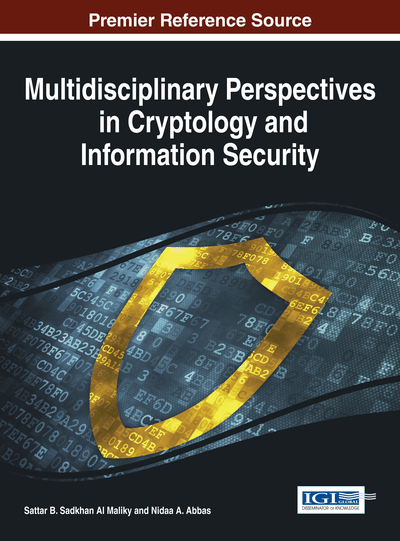# Cryptography Based on Error Correcting Codes: A Survey

Marek Repka, Pierre-Louis Cayrel
DOI: 10.4018/978-1-4666-5808-0.ch005
OnDemand:
(Individual Chapters)
Available
\$29.50
No Current Special Offers

## Abstract

Breaking contemporary cryptographic algorithms using any binary computer has at least sub-exponential complexity. However, if a quantum computer was used effectively, then our asymmetric cryptography would not be secure anymore. Since the code-based cryptography (cryptography based on error-correcting codes) relies on different problems, it is not as threatened as, for example, RSA or ECC. Recent years have been crucial in the progress of cryptography based on error-correcting codes. In contrast to the number-theoretic problems typically used in cryptography nowadays, certain instances of the underlying problems of code-based cryptography remain unbroken even employing quantum cryptanalysis. Thus, some code-based cryptography constructions belong to the post-quantum cryptography, especially cryptosystems based on binary irreducible Goppa codes. Many attempts to replace this underlying code in order to reduce the key size already have been proposed. Unfortunately, almost all of them have been broken. For instance, just a while ago, Reed Muller, Generalized Reed-Solomon Codes, and Convolutional codes were broken. Against some rank metric codes, a new attack was introduced. On the other hand, two prospective countermeasures in order to hide the exploitable code structure of the broken codes were fashioned. However, only the choice of binary irreducible Goppa codes remains secure in the post-quantum sense. This chapter surveys the more recent developments in code-based cryptography as well as implementations and side channel attacks. This work also recalls briefly the basic ideas, and provides a roadmap to readers.
Chapter Preview
Top

## Introduction

Present asymmetric cryptography is mainly based on discrete logarithm problem, such as cryptosystems like Diffie-Hellman key exchange protocol, DSA, or ElGamal. Elliptic Curve Cryptography (ECC) relies on the hardness of discrete logarithm defined over elliptic curves (ECDLP). Another problem is the integer factorization problem that RSA is based on. Note that the first purpose, the elliptic curves were used in cryptography, was integer factorization. In solving the discrete logarithm or factorization problem, fast progress has been made. For integer factorization, the general number field sieves are used, and for solving the discrete logarithm, the index calculus algorithm can be employed. Even the binary computation power has grown as Moore's law states. We have new integrated circuit technologies, and special devices for cryptanalysis. We have clusters, grids and clouds today. But we still believe that the complexity of present asymmetric cryptography is at least sub-exponential. Since cryptography based on error-correcting codes relies on different problems as the discrete logarithm or integer factorization, it is not threaten by breaking those problems. Furthermore, a more serious problem is that the threat of an effective use of quantum computer has arisen significantly. Clearly, if an adversary is able to use a quantum computer effectively, almost all the asymmetric cryptography algorithms are threatened (Shor, 1997). The Shor’s quantum algorithm (Shor, 1997) complexity is analyzed and improved in (Zalka, 1998). Regarding this quantum algorithm, the discrete logarithm problem as well as the integer factorization problem has polynomial complexity, even on a quantum computer. The case of ECDLP quantum cryptanalysis is investigated more deeply in (Proos, & Zalka, 2003). In certain cases of the error-correcting code-based cryptography, there is no better than a sub-exponential quantum attack (Bernstein, 2010).

As mentioned above, the code-based cryptography is based on different problems as usually used today. This, in certain cases, gives the code-based cryptography a feature that is called quantum attack resistance. Quantum attack resistance means that the problem a cryptographic primitive is based on is NP-complete, thus at least sub-exponential, to solve on binary and quantum computer. Such cryptography is called post-quantum cryptography. Note that another possibility how post-quantum cryptography can be constructed is to use lattices, multivariate quadratic equations, or hash functions. More about post-quantum cryptography can be found in the book of Bernstein, Buchmann, and Dahmen (2008).

Yes, post-quantum cryptography is unbreakable today. But, despite the post-quantum cryptography being very resistant to known attacks, problems can arise when such strong cryptographic algorithms, like McEliece PKC (McEliece, 1978) or Niederreiter PKC (Niederreiter, 1986), are implemented in real devices, and post-quantum cryptography is not an exception. By this, we are pointing out the Side-Channel Attacks, which we summarize in the Section Cryptanalysis.

In this work, we are focused on the progress in the last three years particularly, thus we refer a reader that is interested in the earlier work in this field to the (Overbeck, & Sendrier, 2008) or Cayrel et al. (2011) surveys.

## Complete Chapter List

Search this Book:
Reset